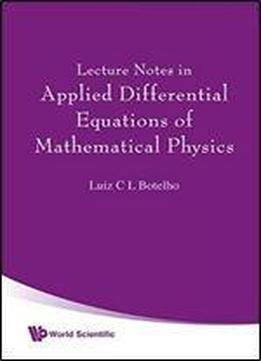# Lecture Notes In Applied Differential Equations Of Mathematical Physics 2008 / English / PDF

Functional analysis is a well-established powerful method in mathematical physics, especially those mathematical methods used in modern non-perturbative quantum field theory and statistical turbulence. This book presents a unique, modern treatment of solutions to fractional random differential equations in mathematical physics. It follows an analytic approach in applied functional analysis for functional integration in quantum physics and stochastic Langevin-turbulent partial differential equations.

views: 185